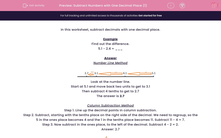# Subtract Numbers with One Decimal Place (1)

In this worksheet, students subtract a mix of whole numbers decimals with one decimal place. Two methods are shown.Key stage:  KS 2

Curriculum topic:   Maths and Numerical Reasoning

Curriculum subtopic:   Decimals

Difficulty level:#### Worksheet Overview

In this worksheet, subtract decimals with one decimal place.

Example

Find out the difference.

5.1 - 2.4 = ___

Number Line MethodLook at the number line.

Start at 5.1 and move back two units to get to 3.1

Then subtract 4 tenths to get to 2.7

Column Subtraction Method

Step 1. Line up the decimal points in column subtraction.
Step 2. Subtract, starting with the tenths place on the right side of the decimal. We need to regroup, so the 5 in the ones place becomes 4 and the 1 in the tenths place becomes 11. Subtract 11 - 4 = 7.
Step 3. Now subtract in the ones place, to the left of the decimal. Subtract 4 - 2 = 2.